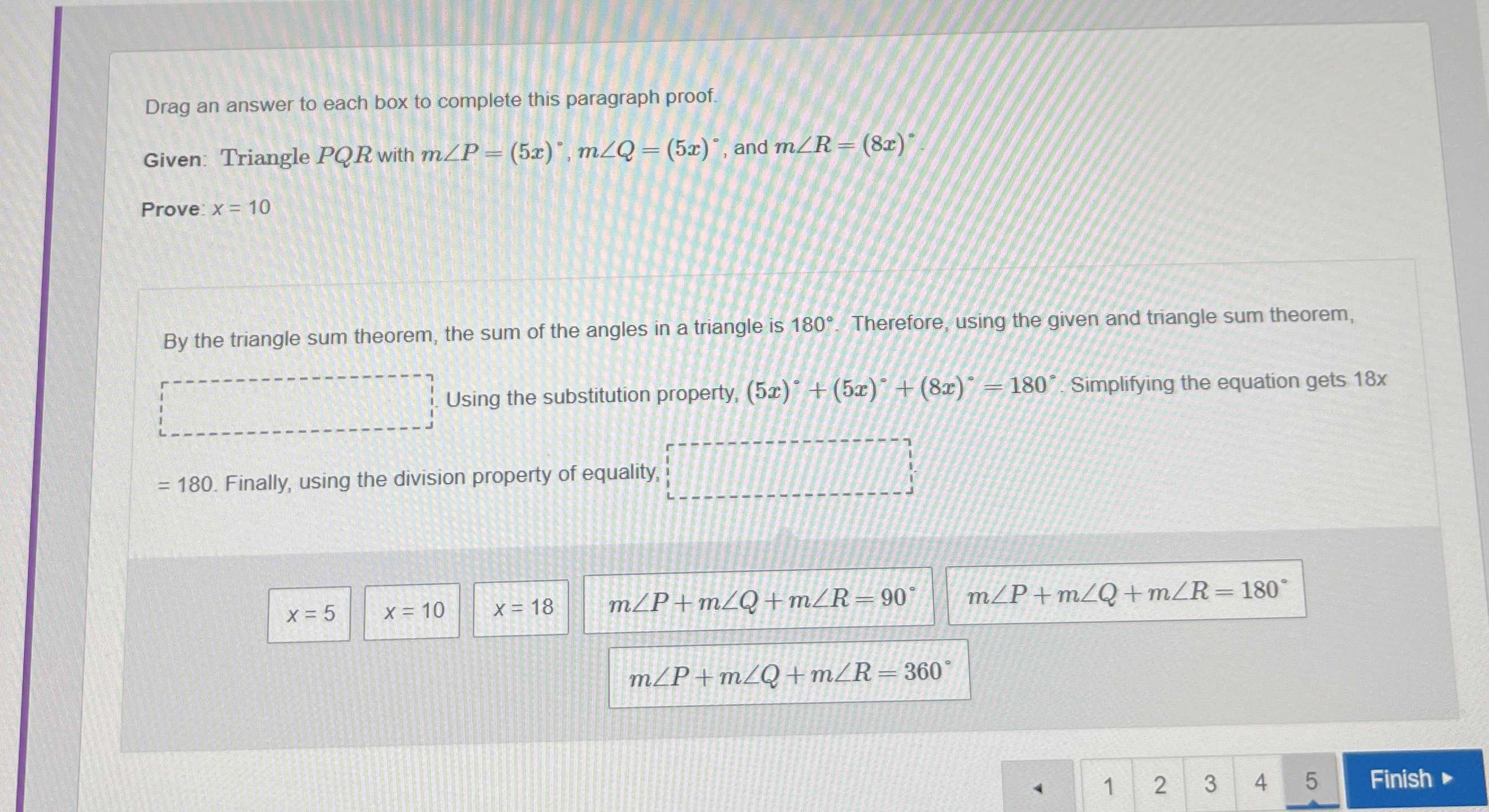### Still have math questions?

Trigonometry
QuestionBy the triangle sum theorem, the sum of the angles in a triangle is $$180 ^ { \circ }$$ . Therefore, using the given and triangle sum theorem, $$\square$$ Using the substitution property, $$( 5 x ) ^ { \circ } + ( 5 x ) ^ { \circ } + ( 8 x ) ^ { \circ } = 180 ^ { \circ }$$ Simplifying the equation gets $$18 x= 180$$ . Finally, using the division property of equality, $$\square$$

$$x = 5$$   $$x = 10$$  $$x = 18$$$$m \angle P + m \angle Q + m \angle R = 90 ^ { \circ } \quad m \angle P + m \angle Q + m \angle R = 180 ^ { \circ }$$$$m \angle P + m \angle Q + m \angle R = 360 ^ { \circ }$$

$$m\angle P+ m\angle Q+ m\angle R= 180°$$